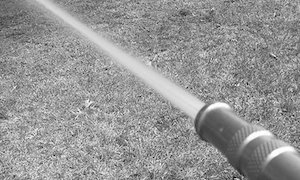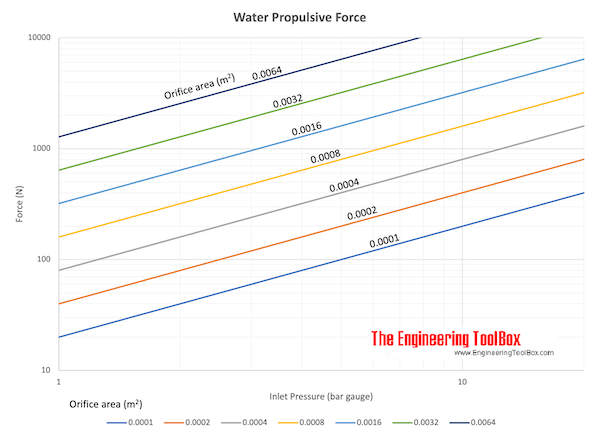Engineering ToolBox - Resources, Tools and Basic Information for Engineering and Design of Technical Applications!

# Jet Propulsion

## Calculate the propulsive discharge force or thrust induced by an incompressible jet flow.

The velocity out of a free jet can be expressed as

v2 = (2 (p1 - p2) / ρ)1/2                              (1)

where

v2 = velocity out of the jet (m/s)

p1 = pressure before the jet (N/m2, Pa)

p2 = ambient pressure after the jet (N/m2, Pa)

ρ = density of the fluid (kg/m3)

Note! - the density ρ is constant in incompressible flows and the equations are valid for liquids (like water) but not for gases (like air).

The flow volume out of a jet can be expressed as

q = A v2                                (2)

where

q = volume flow (m3/s)

A = orifice area of the jet (m2)

The propulsive force or thrust induced by the jet can be expressed as

F = ρ q (v2 - v1)                                (3)

where

v1 = jet velocity (m/s)

If the jet is not moving

v1 = 0

and (3) can be expressed as

F = ρ q v2                                    (3b)

- or alternatively with (2)

F = ρ A v22                                  (3c)

Substituting v2 with eq. (1) in eq. (3c) - the propulsive force or thrust induced by a jet not moving (v1 = 0) be expressed as

F = 2 A (p1 - p2)                                 (4)

### Example - Propulsive ForceWater flows through a garden hose with diameter 15 mm. The water pressure just before the outlet is 4 105 Pa (abs) and the atmospheric pressure is 1 105 Pa (abs).

The outlet area of the hose can be calculated as

A = π ((15 mm) (0.001 m/mm) / 2)2

= 1.77 10-4  (m2)

The propulsive force can be calculated as

F = 2 (1.77 10-4 m2) ((4 105 Pa) - (1 105 Pa))

= 106 N## Related Topics

• ### Fluid Mechanics

The study of fluids - liquids and gases. Involving velocity, pressure, density and temperature as functions of space and time.

## Related Documents

• ### Force

Newton's third law - force vs. mass and acceleration.
• ### Hose - Water Discharge Volume Flow vs. Pressure

Water discharge through hoses - pressure ranging 10 - 200 psi (0.75 - 14 bar).
• ### Hoses - Pressure Loss vs. Water Flow

Pressure loss in hoses due to water flow and friction.
• ### Impact Force

Impact forces acting on falling objects hitting the ground, cars crashing and similar cases.
• ### Impulse and Impulse Force

Forces acting a very short time are called impulse forces.
• ### Open Horizontal Pipes - Discharge Length vs. Volume Flow

Discharge length vs. volume flow from water filled horizontal pipes.
• ### Orifice, Nozzle and Venturi Flow Rate Meters

The orifice, nozzle and venturi flow rate meters makes the use of the Bernoulli Equation to calculate fluid flow rate using pressure difference through obstructions in the flow.
• ### Piping Elbows - Thrust Block Forces

Thrust block forces on pipe bends anchors due to liquid velocities and internal pressures - online resulting force calculator.
• ### Vertical Pipes - Discharge Height vs. Volume Flow

Volume flow and discharge height from vertical water pipes.

## Engineering ToolBox - SketchUp Extension - Online 3D modeling!

Add standard and customized parametric components - like flange beams, lumbers, piping, stairs and more - to your Sketchup model with the Engineering ToolBox - SketchUp Extension - enabled for use with older versions of the amazing SketchUp Make and the newer "up to date" SketchUp Pro . Add the Engineering ToolBox extension to your SketchUp Make/Pro from the Extension Warehouse !

We don't collect information from our users. More about

## Citation

• The Engineering ToolBox (2013). Jet Propulsion. [online] Available at: https://www.engineeringtoolbox.com/jet-discharge-propulsion-force-d_1868.html [Accessed Day Month Year].

Modify the access date according your visit.

9.19.12